# GeoMaestro

-- Back --

## Projectors: from events to MIDI phrases

Principles
Fundamental functions: `Ecoute()` and `EcouteC()`
The `Volume[]`, `Pit[]`, `Pan[]` and `Dur[]` arrays of functions
The `Time[]`, `Mer[]` and `CScore[]` arrays of functions
Projectors

Projectors reference page

## 1: Principles

(...also see this paper for an informal overview)

Here are five events that could have been generated with the graphic tool (this image was actually taken from a screen snapshot):To get a musical phrase from these static events, we have to define first when each of them will be played. To do so, we give GeoMaestro a support (segment or circle) to project them onto.

Here are two segments we can use: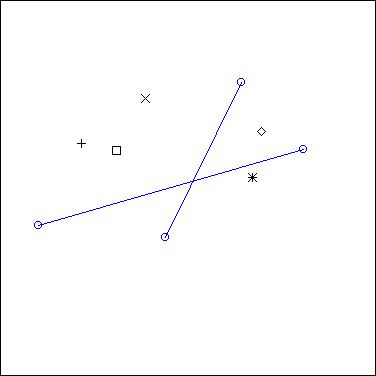Projection on the longest one is the operation represented below by red lines: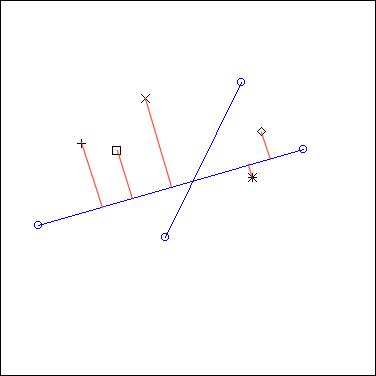... and here is projection on the other one: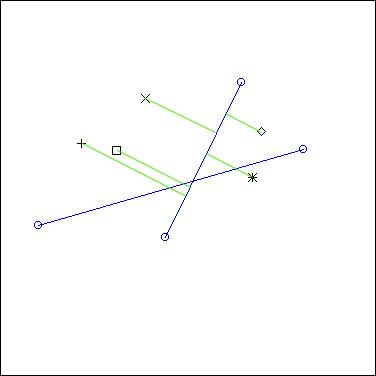As you can see, projection means actually orthogonal projection (that is: a right angle is used to get the projecting line from the segment to the event)

Now, this operation only gives the order and the respective time values of the notes in the eventual phrase (once we have chosen whether the segment is going to be read from right to left or from left to right) . There is much more to define: durations of notes, pitchs, panning, velocities. The event's nodur ("nodur" stands for "note and duration", but it can actually be any KeyKit phrase) contains such information, but it's only here to be changed (or not) !

So these values are not automatically calculated, since GeoMaestro is intended to let the composer free to compose. Instead, we will define special functions which take as parameters the distance, angle and side from the event to the segment, and of course the event nodur (note value) to calculate the different note attributes. Some of theses functions are already here, ready to use, but you are free and welcome to write your own.

Before we go on and see that in details, here are three other examples when a circle is used for projection: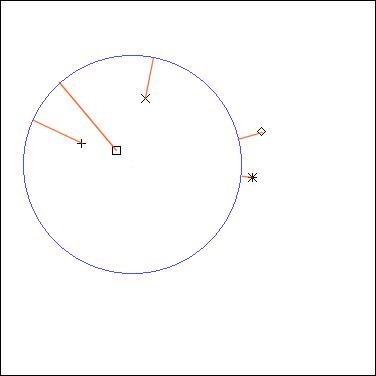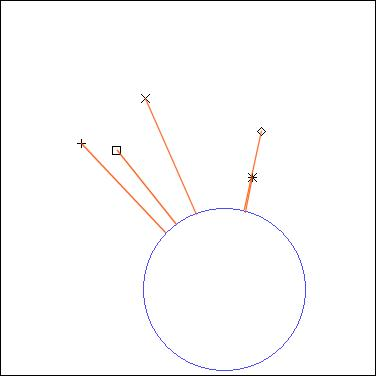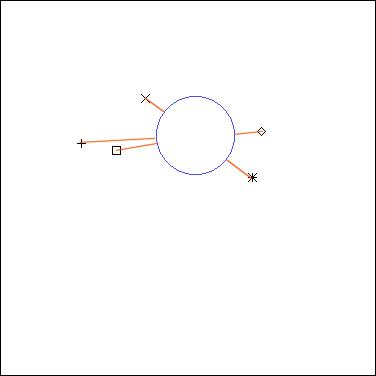... it's pretty much the same principle as with the segment projection, apart from the fact that you need to define one more thing here: the starting point of the rendered phrase.

So you can see that this simple set of five events can very easily be "listened to" in a large variety of ways.

## 2: Fundamental functions: `Ecoute()` and `EcouteC()`

("ecoute" is French for "listen to")

The `Ecoute()` and `EcouteC()` functions are the implementations of the principle we just saw before. Their syntax can be very simple:
`L = Ecoute(A, B)`
... is expecting that `A` and `B` are points (like ["x"= 1, "y"= 2] ). Projection is done onto the segment AB, with `A` being the starting point. Ecoute() returns a ligne L ("ligne" is French for "line", I'll keep it in French here to remind you that it is a specific data structure). The rendered phrase is stored in `L["ph"]`

For a projection on a circle, here's the basic syntax:
`L = EcouteC(C, A, n)`
... were `C` is a circle (like `["c"= ["x"= 0, "y"= 0], "r"= 1]` ), `A` is a point and `n` is an integer.

`A` is used to define the starting point for rendering (we draw the line defined by the center of the circle to `A`, and start rendering at the point were it cuts the circle. If you prefer, you can replace `A` by an angle value: a float from 0 to 2*Pi).
`n` is the number of rounds we do. Usually it's 1 (since in most cases you can simply repeat the rendered phrase if you want more of it; this is not true for events with scripts, though), but it is also used to define the sense of rotation: 1 goes clockwise, and -1 goes counter-clockwise.

You may wonder how the duration of the phrase is calculated. Good question !

By default, it is the length of the support multiplied by the global variable `CPCM`. CPCM stands for "Clicks per CentiMeter", but it actually means "Clicks per GeoMaestro Unit". If you have a look at the initialisations.k file, you'll see:
`CPCM = seconds(1)`
which means that, by default, one unit of length is corresponding to one second. You can change that. (Note that this supposes you're not going to change the tempo: see here for more)

Now you may want to set the length of the phrase rendered by `Ecoute()` or `EcouteC()`. To do so, you need to give it one more argument:
`Ecoute(A, B, duration) EcouteC(C, A, n, duration)`
Here `duration` is an integer, with a very specific use. Let's say that the support's length is `length`, and the duration of the rendered phrase is `phdur`:

if `duration` is 0, we stick to the default:
`phdur = CPCM*length`

if `duration` < 0, we are in relative mode:
`phdur = |duration|*CPCM*length`

if `duration` > 0, we are in absolute mode:
`phdur = duration`

Remember that the duration unit if the click. To know how many clicks you have in a second, type ` print seconds(1) ` in the console (See KeyKit help files). So in absolute mode, duration is a number of clicks and it will be the length of the phrase, independently of the length of the support. You can see the interest of this mode in the `CercleRythm()` projector, for example.

Note that `duration = 0` and `duration = -1` give the same results: the default relative mode.
`duration = -2` makes the "tempo" get divided by 2, while `duration = -0.5` makes it double.

The two next optional arguments are: the starting time `t0` of the phrase (by default it's 0), and the `region` of the event scene we're listening to (by default, it's `Tout`, that is: everything); events that do not belong to the region will be ignored.

So the complete syntax with all parameters introduced so far is:
`Ecoute(A, B, duration, t0, region) EcouteC(C, A, n, duration, t0, region)`

If you don't know what a region is, go and read the data structures documentation. Also see here

There is a last optional parameter: `df`; we will see it later on, after an overview of the distortion functions arrays.

Note (which you can skip at first): any one of the optional parameters can be replaced by a closure ID. It can also be given as an extra parameter, after `df`. More about this here.

## 3: The `Volume[]`, `Pit[]`, `Pan[]` and `Dur[]` arrays of functions

Now we will have a look at what's happening inside the `Ecoute()` and `EcouteC()` functions.

To decide how events are going to become notes when projected on a support, specific functions are used for each note attribute. They are contained in arrays because they are also specific to channels.

The function arrays are defined and initialized in the initialisations.k file. This is where you can set their default values.
The functions themselves have their code written in the lib_dist.k file, and that's were you can add your home-made ones, in such a way that they will be smoothly integrated to the GUI tool (see advanced features for more).

So here's the idea: each projected event is associated with a distance and an angle (to the support), and also with a side (it comes from left or right). In the following picture, distance from the event to the support (segment AB) is represented in red, angle is grossly -Pi/4 (that is 7*Pi/4), and side is 1 (right) if the support is read from A to B, -1 (left) if it is read from B to A: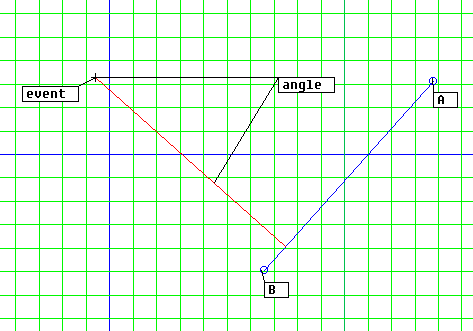Each event also contains a nodur, that is a note or a phrase. So these four elements are the arguments we will use. A fifth argument is the channel number, which can be useful in many ways (for example when using channel-specific parameters).

Note: there is also a last argument, not used so far by any function in the distribution: the local time `t`; its usage is tricky because its value depends on the kind of projector the support belongs to; check tutorial 11 for an example of usage. Let's forget about it for now.

Let's see all this with an example:

suppose we have
```Volume = "Vexp1"
Pit = "NoChanges"
Dur= "NoChanges"
Pan= "BasicPan"```
... this will completely define how is treated an event belonging to channel 5. The string values are the names of the used functions (we use KeyKit's `efunction()` to convert string to function)

Let's say the event's nodur is `ph`, its distance to the support is `d`, the projection angle `theta` and the side is `s` (`s` is `-1` for left or `+1` for right)

The corresponding note `N` on the rendered phrase will be initially set to `ph` (so it's identical to the event nodur), then have its attributes modified this way:
```N.pitch += NoChanges(ph, s, d, ch, theta)
N.dur += NoChanges(ph, s, d, ch, theta)
N.vol += Vexp1(ph, s, d, ch, theta)
```

Also, a pan message will be send for channel 5, setting the pan to `BasicPan(ph, s, d, ch, theta)`

What does that mean ? We have to look at the codes for these functions, in file lib/lib_dist_old.k. Their names may be a bit weird, especially because they're an horrible mixure of Strange French and Broken English. But don't forget you're supposed to write your own functions ! The ones I provided here are very basic.
(by the way, the lib/lib_dist_old.k file contains code for the distortions functions from the first distributions of GeoMaestro, for versions before 1.066; they all use only global parameters. We wil see a bit later how local parameters can also be managed now, making the usage of such functions much more flexible).

So in lib/lib_dist_old.k we see:
```function Vexp1(ph, s, d) {  return (ph.vol*(exp(-d)-1))  }
function NoChanges() { return (0) }
function BasicPan(ph,s, d) {return (64 + s*integer(Minimum(PAN_FDIST*d,63))) }```
... so we see that `NoChanges()` is judiciously named, since it has no effect ! In this example, both duration and pitch are the same for the event nodur as for the corresponding note.

The volume is affected, though: you can see that an event very close to the support keeps its initial volume, while it gets down very fast if the distance increase. (Note that there is a global variable `SILENCE` set in initialisations.k that defines the volume threshold for keeping the note. By default it is set to 10, which means that an event whose projected note has a volume less than 10 will be considered as silent and ignored).

The pan value is calculated so that a left-hand event will sound at the left hear, while a right-hand event will sound at the right. Also, precise pan depends on the distance so that close events are in the middle of the pan area. The function use a global variable `PAN_FDIST` as a factor to the distance. As for any global variable parameter related to distortion functions, you can set this value in the `InitParameters()` function in the lib_dist.k file.

So the overall effect of these example settings is that you hear events as if you were walking along the support: you hear them at the right or at the left, depending on where they are; and the closest the loudest. The speed of your "walk" would be set with the duration argument in the `Ecoute()` or `EcouteC()` function.

This is only a very basic example... you can easily create huge distortions and define very weird ways of "walking" through an event scene by defining your own functions and mixing their effects.

(Note that the GUI provides three mouse modes ("show distortions", "hear distortion" and "radial distortion") useful to check the distortion functions effect on a specific event. See also the DistMap plug-in for yet another point of view on distortions)

In the example we just saw, all functions depended only upon global parameters (such as `PAN_FDIST`). This is a big limitation, since it means that you can not adjust the distortion effect on a per-channel base.

Since GeoMaestro v1.066, each distortion functions array (`Volume, Pit, Pan, Dur, ``Time, Mer, CScore`) has an associated array storing arguments for local parameters inside the function. A set of arguments is thus available for each channel.

For example, instead of using `BasicPan()` which relies on the single global parameter `PAN_FDIST`, we can use `PanWithDist()`, which does the same thing:
```function PanWithDist(ph, side, dist, ch, tht, t, period)
{
return (64 + side*integer(Minimum(dist/float(period), 63)))
}```
... here the parameter `period` replaces `PAN_FDIST`, so that we can have different Pan scales according to the channel.

The argument lists arrays are:
```VolumeArgs
PitArgs
DurArgs
PanArgs
TimeArgs
MerArgs
CScoreArgs```
To set their values, you can use the `GetArguments()` function (it is the same as the KeyKit `argvlist()` function, which transforms function arguments into an array: see KeyKit documentation for details).

For example,
`VolumeArgs = GetArguments(10, 100, 1000)`
does the same as
`VolumeArgs = [0=10, 1=100, 2=1000]`
... it means that the Volume distortion function for channel 8 will be called with the following arguments:
`(ph, s, d, 8, theta, t, 10, 100, 1000)`
To be precise: internally, the following call is performed:
`efunction(Volume[ch])(ph, s, d, ch, theta, t, varg(VolumeArgs[ch]))`

This may seem a bit complicated, but that's because I'm giving you all the details here. When you set distortion functions from the GUI, all of this is completely transparent and it's actually quite simple. See tutorial 5 for a quiet and peaceful introduction to the usage of distortion functions.

Alternate syntax

To be comprehensive, let me describe here another way to define a distortion function. You may skip this part if you're just discovering the system.

What was described so far implicitly supposed that the functions you want to use for distortions are already defined somewhere (for example in files lib/lib_dist_old.k or lib/lib_dist.k). If you want to create a brand new distortion, say for volume, you have to write the corresponding function first, then register it in the corresponding array `Volume`, for example in `Volume` if it is to effect channel 5. This involves using a text editor and `#include`-ing a file.

A more direct way to do so is to register in the `Volume` array the new function code (as a string) instead of a function name. Only the function body (the part between `{}` brackets) is to be provided. A temporary function will be build around it, taking the following arguments:
`ph,s,d,ch,tht,t,p1,p2,p3,p4,p5,p6,p7,p8,p9,p10`
For example, setting
`Volume = "{return(-ph.vol*p1*d)}"`
is exactly the same as writing a new function
```function VolLinearDecrease(ph, s, d, ch, theta, t, p1)
{
return(-ph.vol*p1*d)
}```
and setting
`Volume = "VolLinearDecrease"`

In both cases, the value for `p1` can be set like this
`VolumeArgs = GetArguments(0.1)`
or
`VolumeArgs = [0=0.1]`

## 3 (continued): The `Time[]`, `Mer[]` and `CScore[]` arrays of functions

The `Time` array applies on the time attribute of the event nodur, in the same way as previously described for `Volume`, `Pit` and `Dur`. You can use it to create a time offset depending on distance and side; note that it is then possible to have notes appear "outside" the support for projection, for example notes with negative time. The length of the rendered phrase (as managed by the KeyKit "+" phrase operator) will still be the support's length, though. See tutorial 5 for more.

The array equivalent to `Volume`, etc... for Csound score is `CScore` (that's the Sco item in the GUI). Csound topics are discussed here.

(note that `CScore` distortion functions do not take exactly the same arguments as the other ones).

The `Mer` array is mostly intended to create distortion effects based on controllers. Precisely, the functions it refers to return phrases which will be merged with the distorted nodur. You can for example program a panning effect directly with the pan controller (`Pan` functions only return a number from 0 to 127, which is then interpreted as a pan message), or transform a one-note nodur into a chord.

Another usage of this array is the `RestorePAC` function: it is introduced here

There is more: if, instead of returning a straight phrase, the `Mer[ch]` function returns an array embedding the phrase according to the following format:
`["ph"= ..phrase.. ]`
... then the phrase will completely replace the nodur. In this case all other distortion functions will have no effect, and the `Mer` function is to MIDI what `CScore` is to Csound scores: a single all-encompassing distortion function.

We could summarize by stating that the `Mer` array can be used to do what can not be done with `Pit, Volume, Dur, Time,` and `Pan`
.
Again, see tutorial 5 for examples.

## 4: The projectors (or projection functions)

Projectors are simply algorithms calling `Ecoute()` and/or `EcouteC()` a number of times, and combining their results in a specific way. They do not calculate any projection by themselves, which makes them easy to write as simple functions.

A few dozens of projectors are provided with the GeoMaestro distribution (they are referenced here); you can have a look at their code in files lib/projection.k and lib/lib_proj.k, so that you get insights for creating your own projectors.

You will also find them in the projector menu of the GUI.

Projectors ask for specific arguments, always followed by optionals arguments of Ecoute() and EcouteC(), that is: `duration`, starting time `t0`, `region` and `df`.

`df` has not been introduced yet. It is an array containing distortion functions references which will override the ones defined in `Volume, Pit, Dur, Pan, Time, Mer` and `CScore`. We could say that using this parameter transfers the control of distortion from the scene to the projector.

The `df` format is as follow: each index corresponding to a GeoMaestro channel is itself an array containing distortion function settings. For example:
`df = [1=["Volume"="Vexp", "VolumeArgs"=[0=1,1=50]]`
... will impose `Vexp()` with arguments 1 and 50 as `Volume` distortion function for channel 1. The value of `Volume` and `VolumeArgs` will be ignored by a projector having `df` as last argument. All other distortion settings will be respected.

The default values for optional arguments are the following global variables (set in lib/initialisations.k), which you can change if you like:
```DefDuration	# initially 0
DefT0		# initially 0
DefRegion	# initially Tout = [0=0]
DefDF		# initially []```

-- Back --